• 2018-07-26 00:05:42

下面这张图是一个简单的线性回归模型,图中标注了每个样本点预测值与真实值的残差。计算 SSE 为多少？A. 3.02

B. 0.75

C. 1.01

D. 0.604

答案：A

解析：SSE 是平方误差之和（Sum of Squared Error），SSE = (-0.2)^2 + (0.4)^2 + (-0.8)^2 + (1.3)^2 + (-0.7)^2 = 3.02

更多相关内容
• 请看下图当中的两个例子：分别求训练误差以及测试误差

请看下图当中的两个例子：分别求训练误差以及测试误差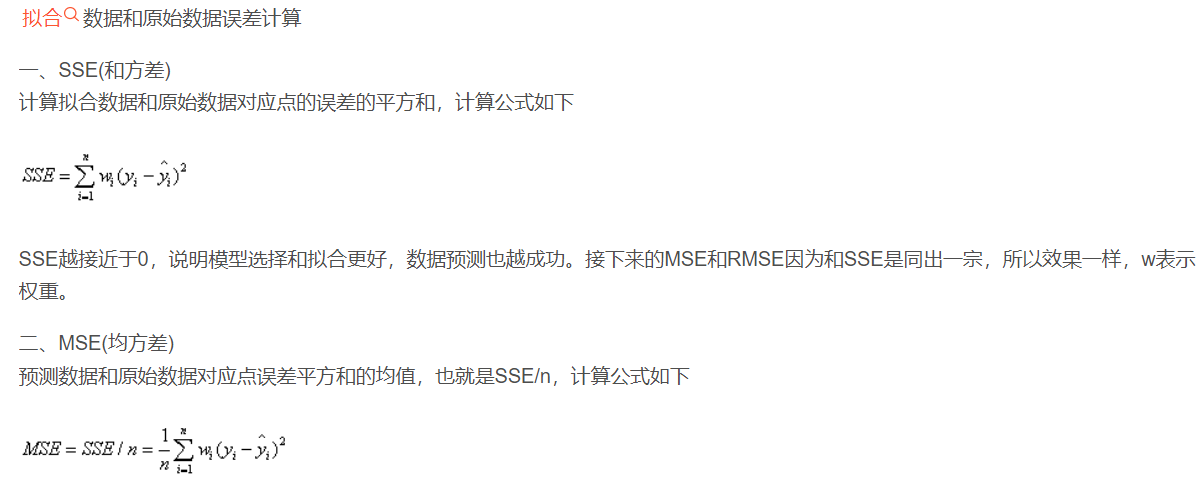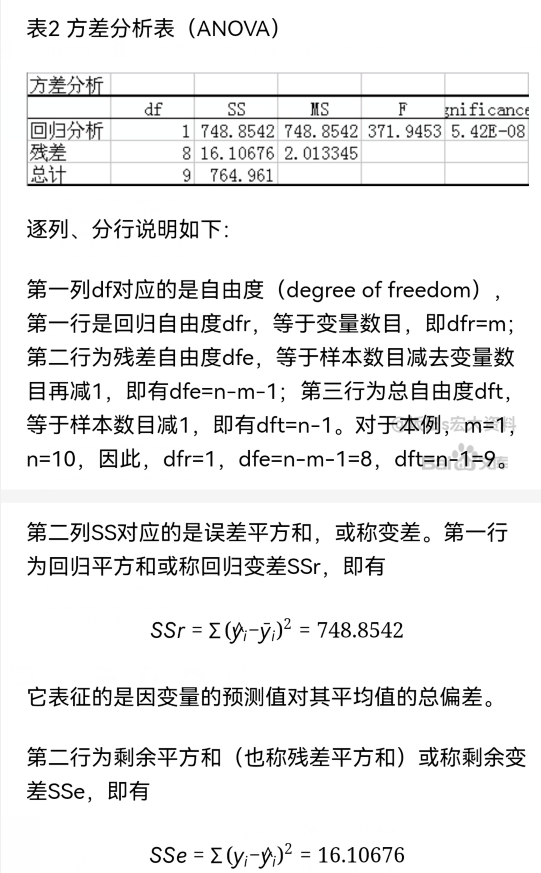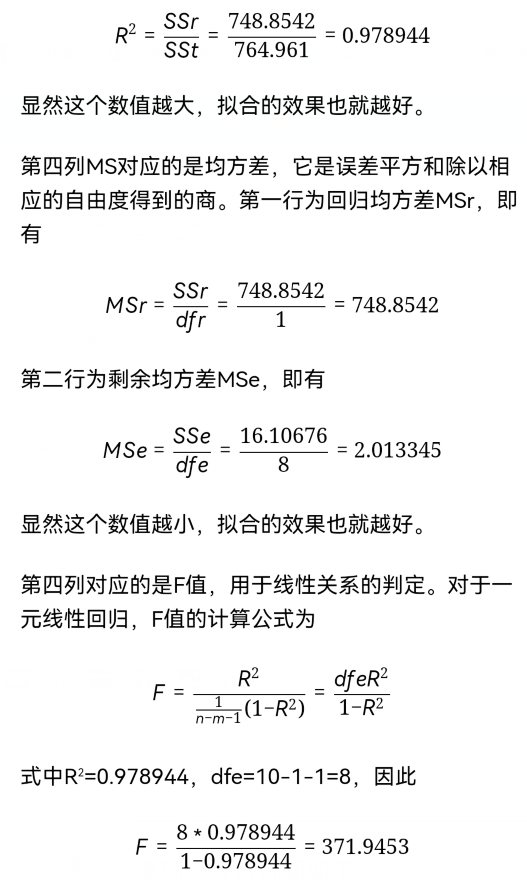##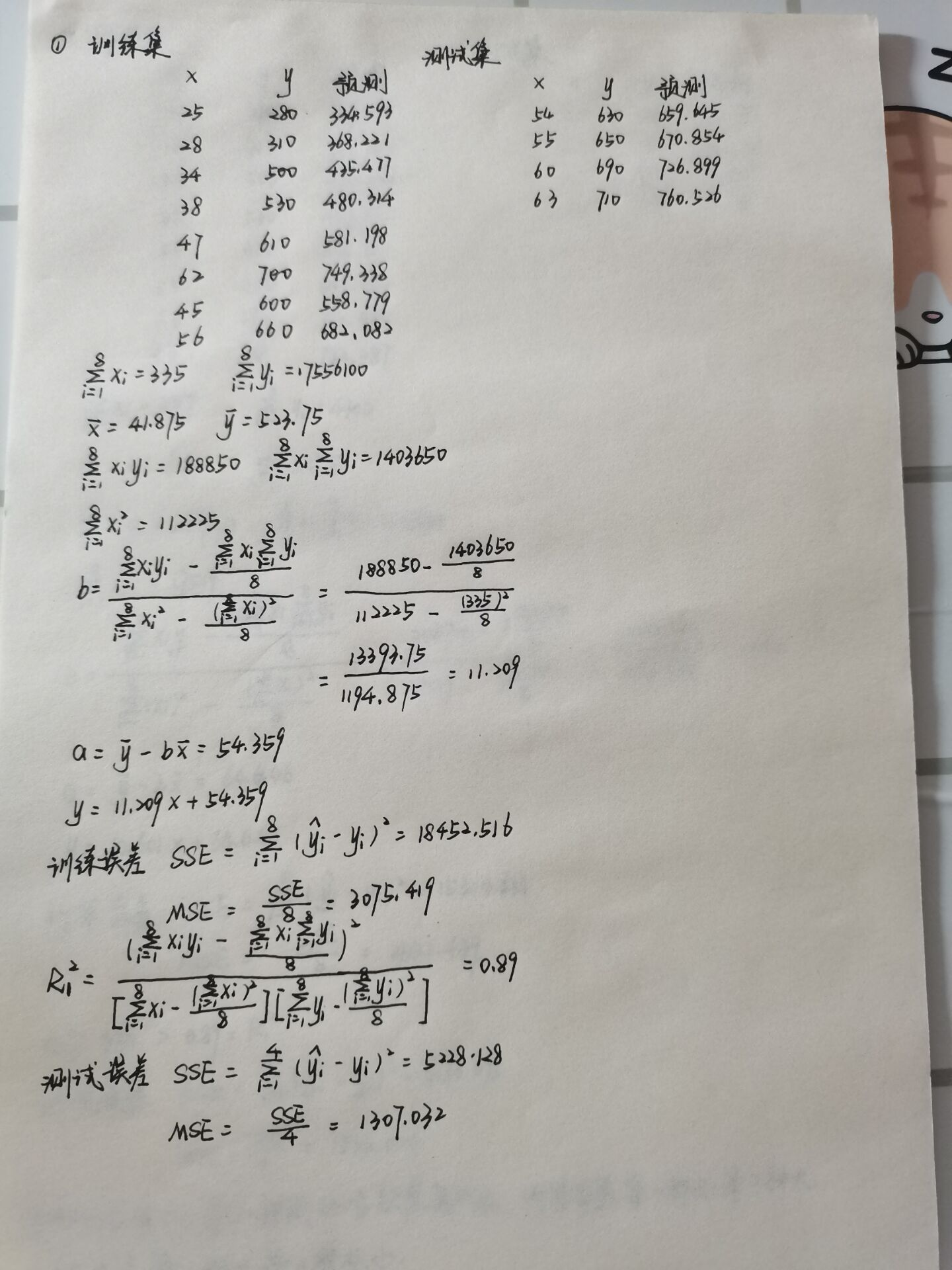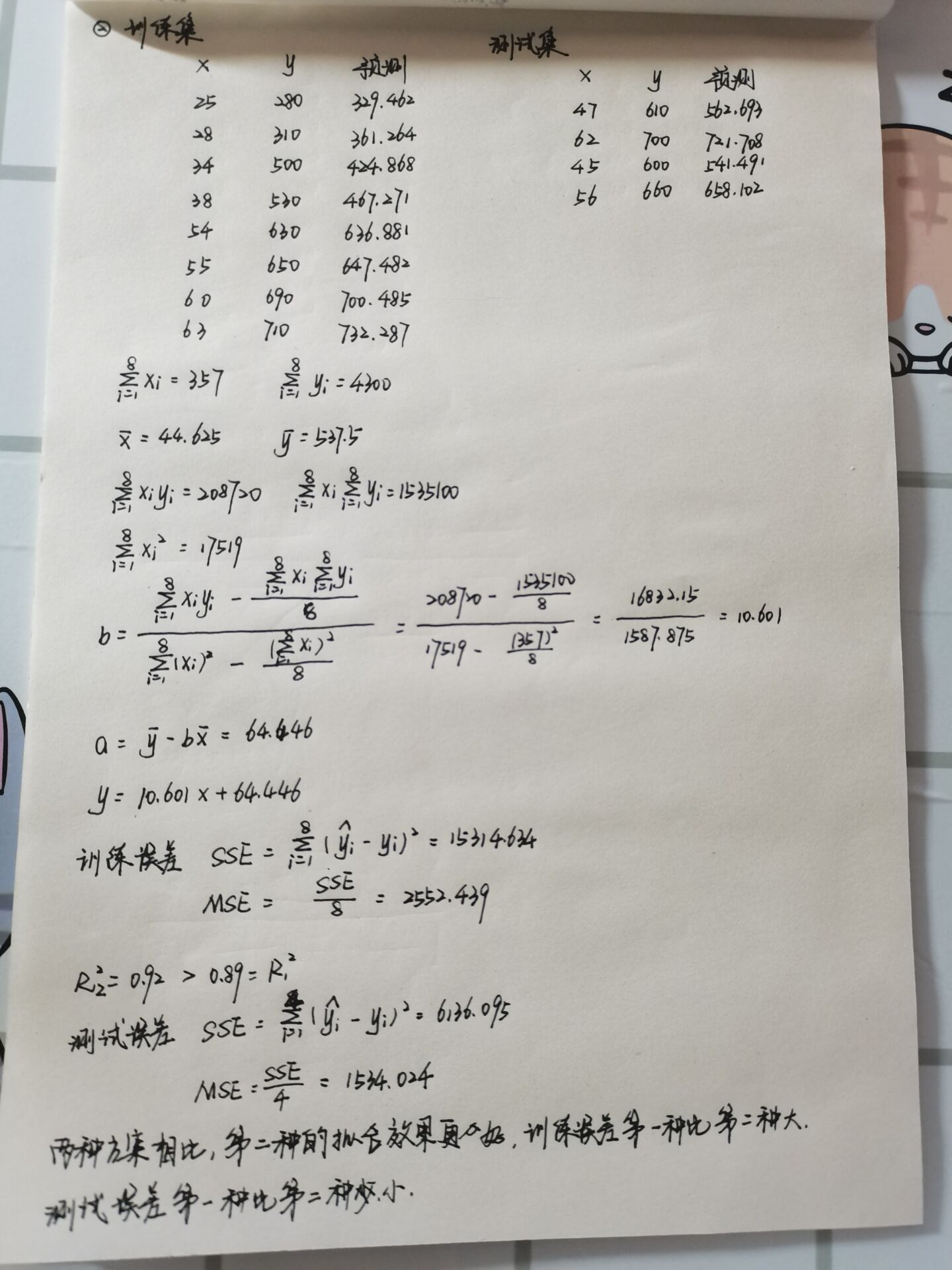决策树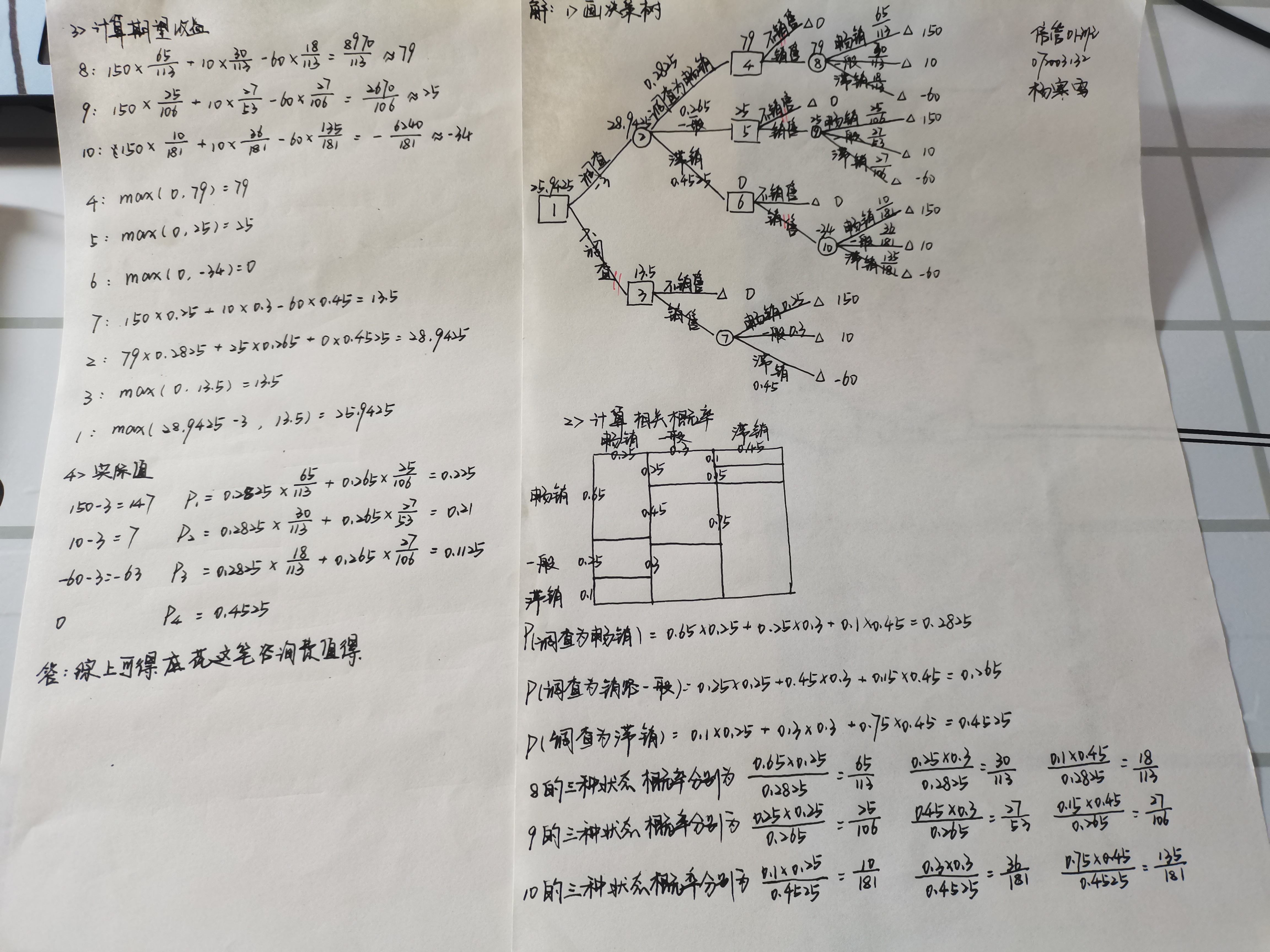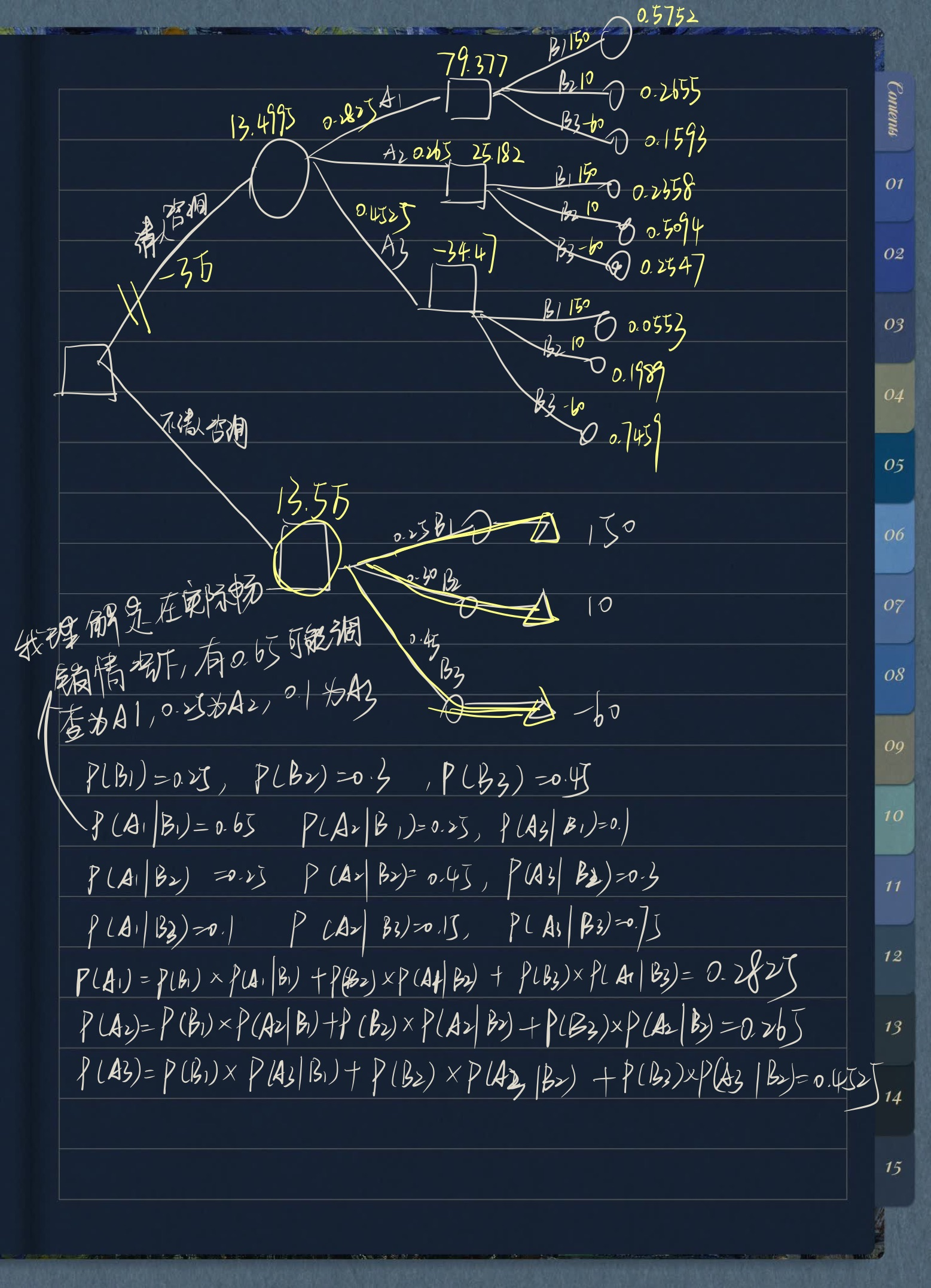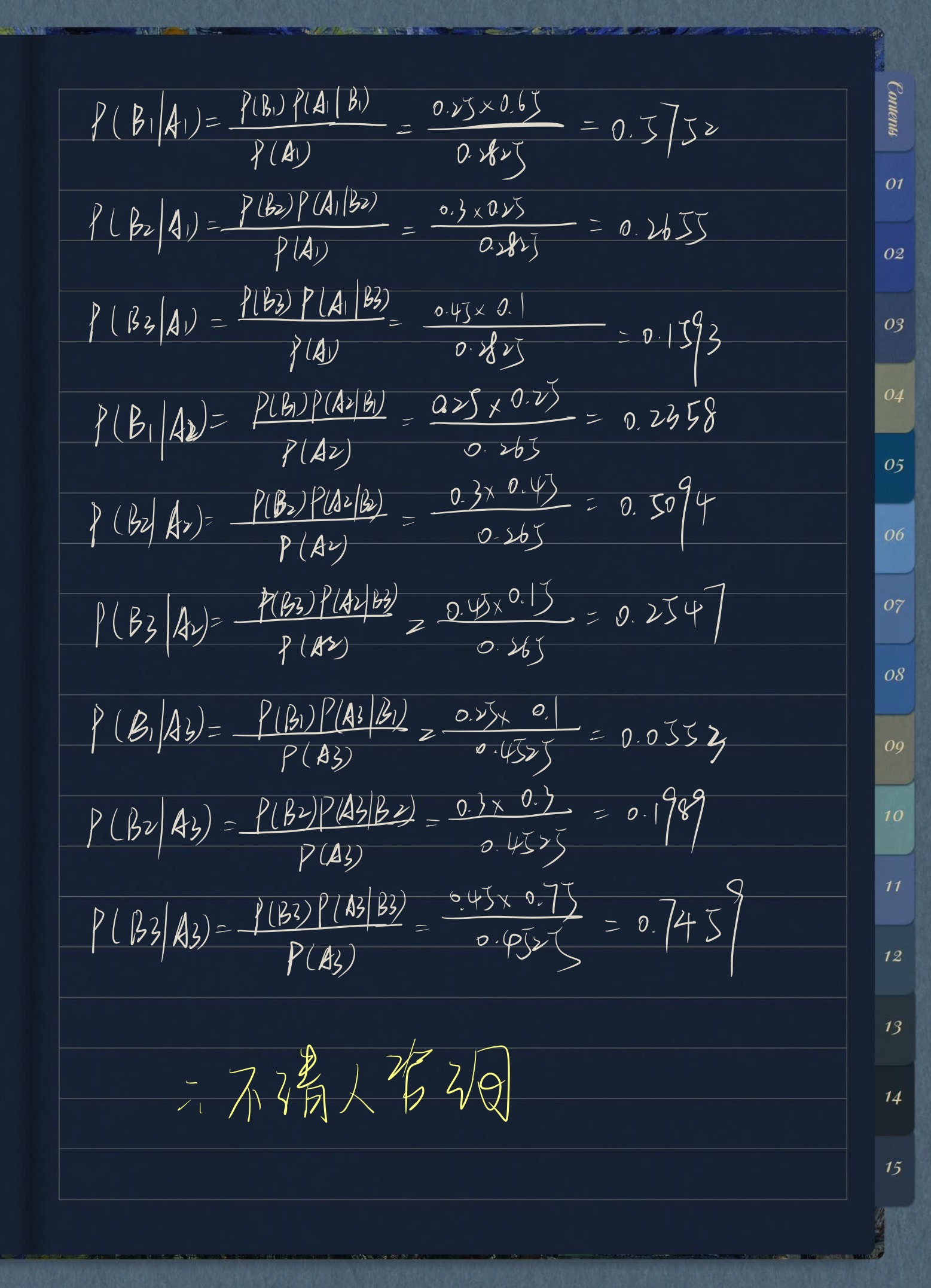展开全文• 模型评估（误差平方和(SSE The sum of squares due to error)）

## 1 误差平方和(SSE The sum of squares due to error)：¶

举例:(下图中数据-0.2, 0.4, -0.8, 1.3, -0.7, 均为真实值和预测值的差)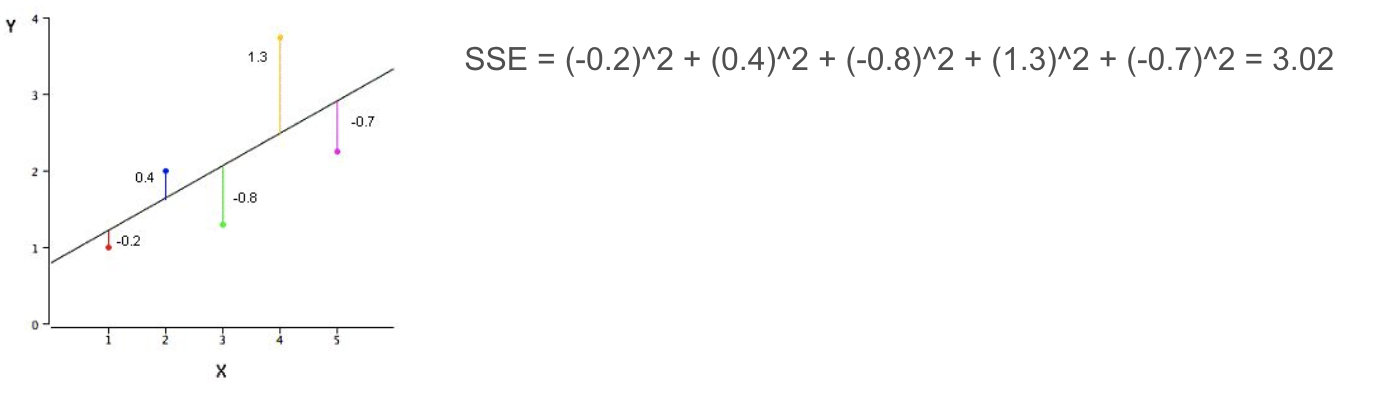在k-means中的应用: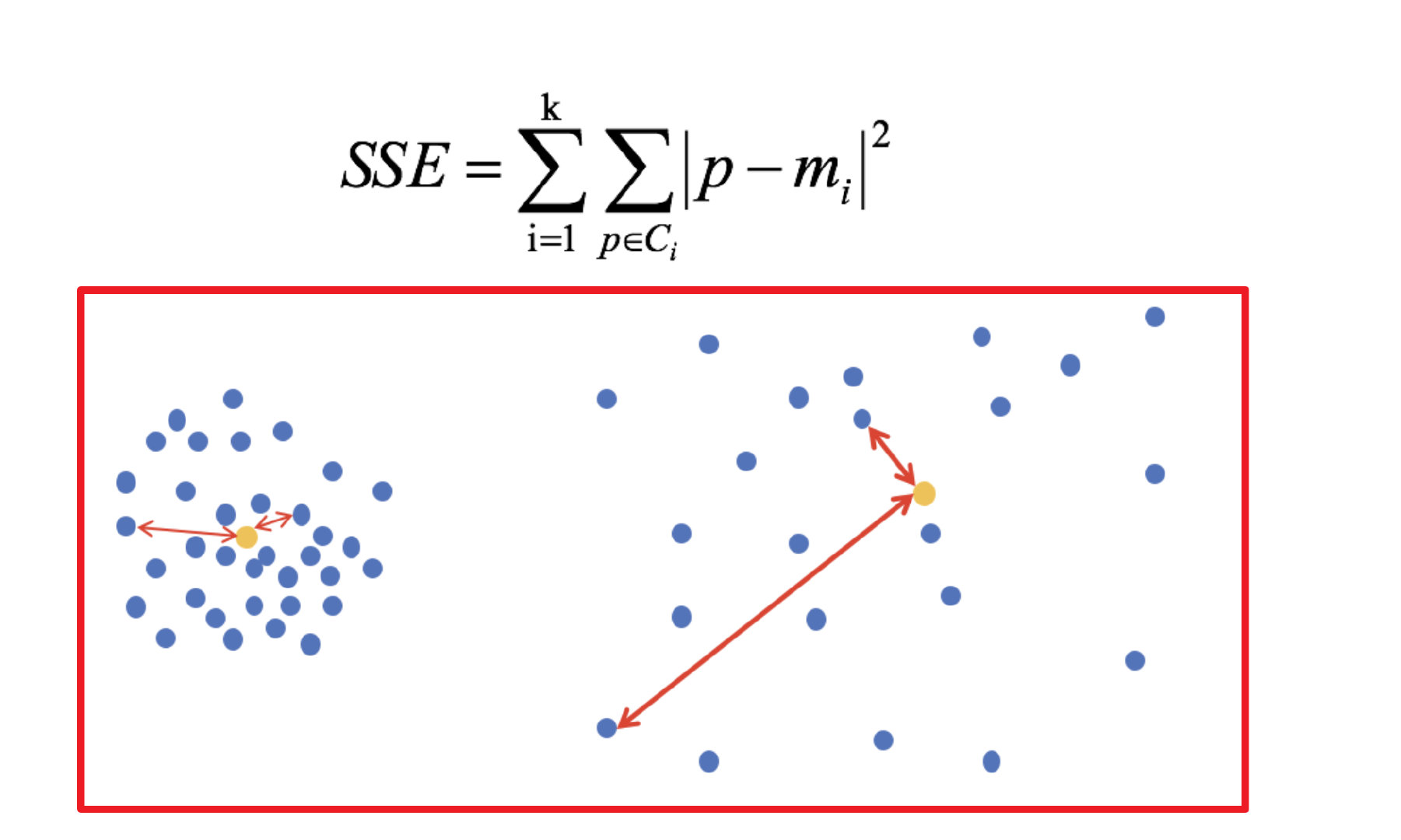公式各部分内容: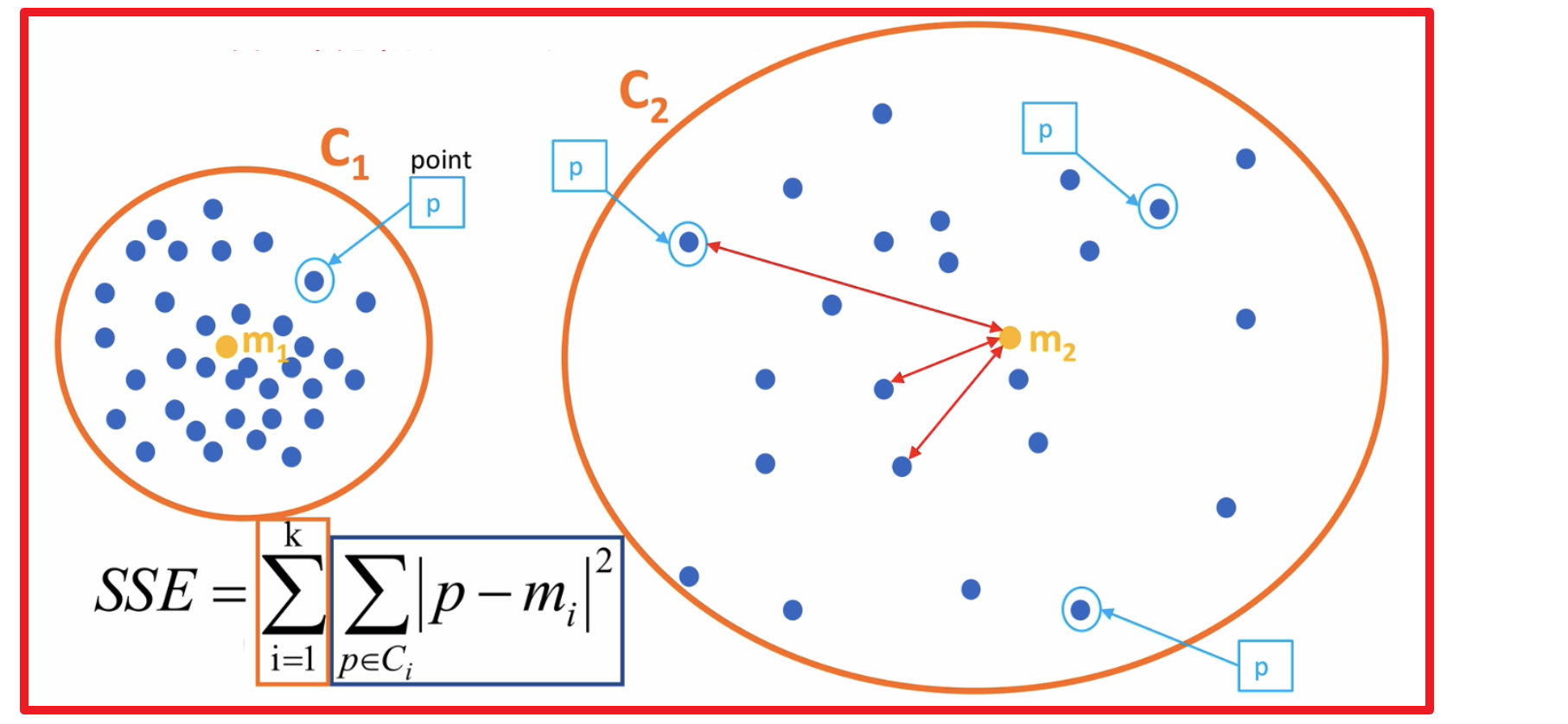上图中: k=2

• SSE图最终的结果,对图松散度的衡量.(eg: SSE(左图)<SSE(右图))

• SSE随着聚类迭代,其值会越来越小,直到最后趋于稳定:

• 如果质心的初始值选择不好,SSE只会达到一个不怎么好的局部最优解.

## 2 “肘”方法 (Elbow method) — K值确定¶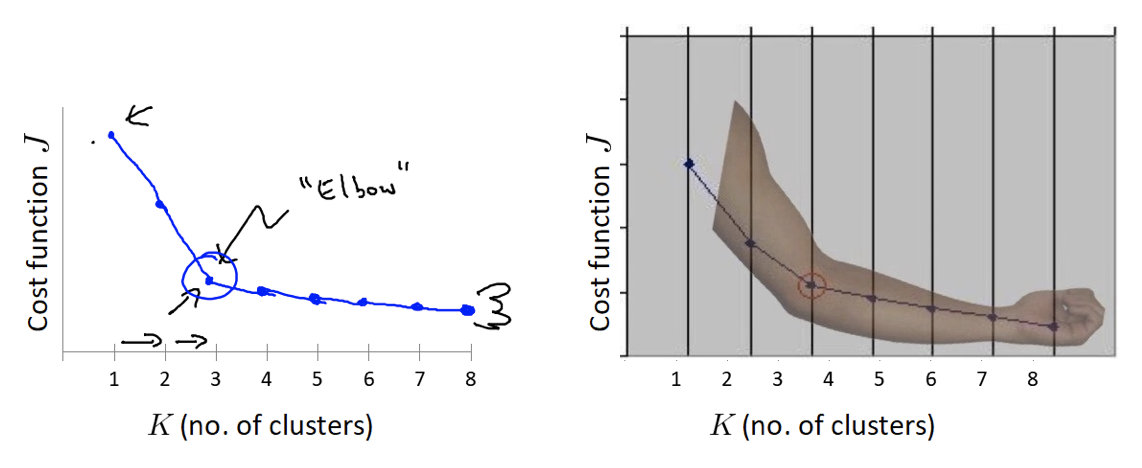（1）对于n个点的数据集，迭代计算k from 1 to n，每次聚类完成后计算每个点到其所属的簇中心的距离的平方和；

（2）平方和是会逐渐变小的，直到k==n时平方和为0，因为每个点都是它所在的簇中心本身。

（3）在这个平方和变化过程中，会出现一个拐点也即“肘”点，下降率突然变缓时即认为是最佳的k值

在决定什么时候停止训练时，肘形判据同样有效，数据通常有更多的噪音，在**增加分类无法带来更多回报时，我们停止增加类别**。

## 3 轮廓系数法（Silhouette Coefficient）¶

结合了聚类的凝聚度（Cohesion）和分离度（Separation），用于评估聚类的效果：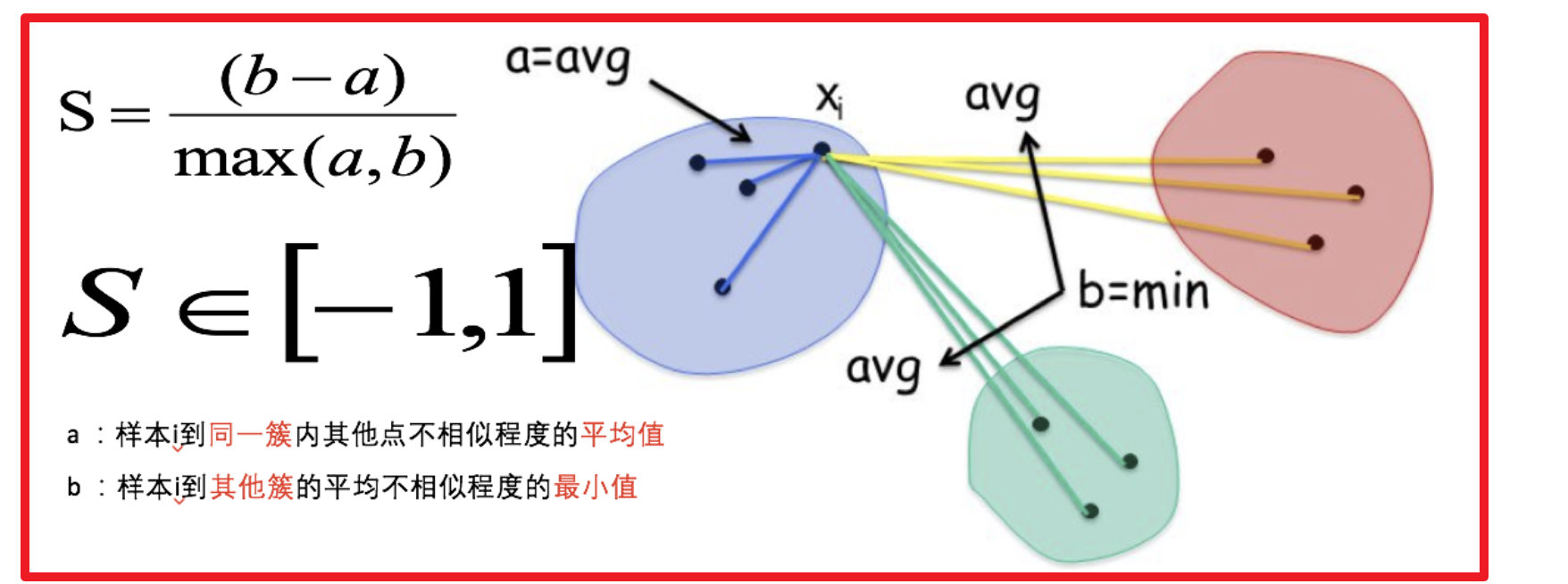目的：

​ 内部距离最小化，外部距离最大化

计算样本i到同簇其他样本的平均距离ai，ai 越小样本i的簇内不相似度越小，说明样本i越应该被聚类到该簇。

计算样本i到最近簇Cj 的所有样本的平均距离bij，称样本i与最近簇Cj 的不相似度，定义为样本i的簇间不相似度：bi =min{bi1, bi2, ..., bik}，bi越大，说明样本i越不属于其他簇。

求出所有样本的轮廓系数后再求平均值就得到了**平均轮廓系数**。

平均轮廓系数的取值范围为[-1,1]，系数越大，聚类效果越好。

簇内样本的距离越近，簇间样本距离越远

案例：

下图是500个样本含有2个feature的数据分布情况，我们对它进行SC系数效果衡量：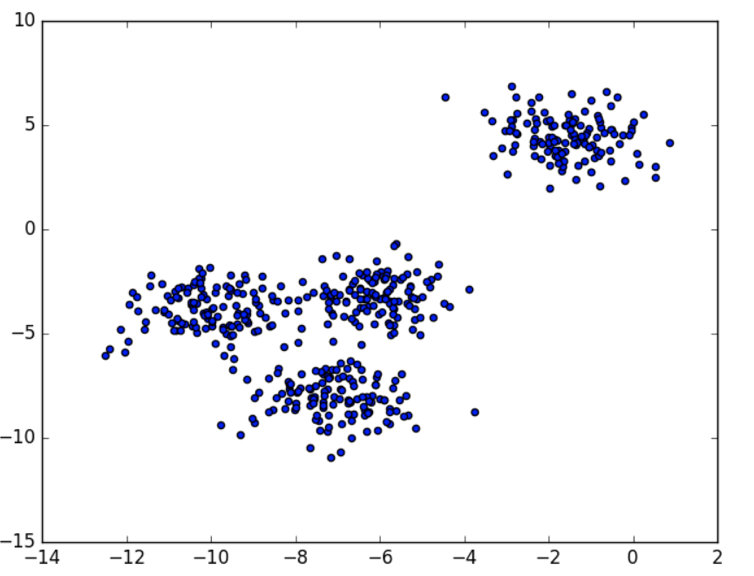n_clusters = 2 The average silhouette_score is : 0.7049787496083262

n_clusters = 3 The average silhouette_score is : 0.5882004012129721

n_clusters = 4 The average silhouette_score is : 0.6505186632729437

n_clusters = 5 The average silhouette_score is : 0.56376469026194

n_clusters = 6 The average silhouette_score is : 0.4504666294372765

n_clusters 分别为 2，3，4，5，6时，SC系数如下，是介于[-1,1]之间的度量指标：

每次聚类后，每个样本都会得到一个轮廓系数，当它为1时，说明这个点与周围簇距离较远，结果非常好，当它为0，说明这个点可能处在两个簇的边界上，当值为负时，暗含该点可能被误分了。

展开全文算法 人工智能 kmeans
• 离差、偏差、残差、误差 关于离差（与平均值的差值） AVERAGE AVERAGE（期望、平均值） AVEDEV AVEDEV（平均差）：Average ...SST（离差平方和） VAR（方差）：Variance STD（标准差）：Standard Devi...

离差、偏差、残差、误差

# 关于离差（与平均值的差值）

## AVERAGE

AVERAGE（期望、平均值）

## AVEDEV

AVEDEV（平均差）：Average Deviation、Mean Deviation

平均差是各个数据与平均数之差的绝对值的和的平均数。

## SST、VAR、STD

SST（离差平方和）

VAR（方差）：Variance

STD（标准差）：Standard Deviation

方差是各个数据与平均数之差的平方的和的平均数。

# 关于残差（与观测值的差值）

## SSE、MSE、RMSE

SSE（残差平方和、和方差、误差平方和）：Sum of Squares due to Error

MSE(均方差、均方误差)：Mean Squared Error

RMSE(均方根误差、标准误差)：Root Mean Squared Error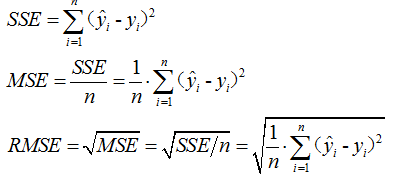# SSE、SSR、SST、R_square

SSE（残差平方和、和方差、误差平方和）：Sum of Squares due to Error

SSR(回归平方和)：Sum of Squares of the Regression

SST(总离差平方和)：Total Sum of Squares

R-square(决定系数)：Coefficient of Determination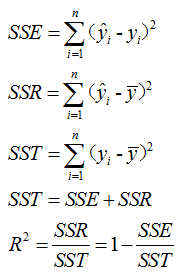R(相关系数)：

R_square(决定系数)：Coefficient of Determination

统计学中的R-square是什么？怎么计算？

展开全文• 误差分析计算公式及matlab代码实现（均方误差MSE，平均绝对误差MAE，平均绝对百分比误差MAPE，均方百分比误差MSPE，均方根误差RMSE，残差平方和SSE
• of_squares(new_K, old_K): ''' 误差平方和 :param data: :param K: :return: ''' return ((old_K - new_K) ** 2).sum() def K_means(dataset, K_point, restart=0): '''标准计算循环完成K-means,误差平方和版本''' ...
• 误差分析计算公式及matlab代码实现（均方误差MSE，平均绝对误差MAE，平均绝对百分比误差MAPE，均方百分比误差MSPE，均方根误差RMSE，残差平方和SSE
• 文章目录聚类算法学习目标6.4 模型评估1 误差平方和(SSE \The sum of squares due to error)：2 **“肘”方法 (Elbow method)** — K值确定3 轮廓系数法（Silhouette Coefficient）4 CH系数（Calinski-Harabasz ...聚类 算法 机器学习 人工智能 数据挖掘
• 证明: 总离差平方和=回归平方和+误差平方和。 SST=SSR+SSE,S S T=S S R+S S E,SST=SSR+SSE, 即 ∑i=1n(yi−yˉ)2=∑i=1n(y^i−yˉ)2+∑i=1n(yi−y^i)2 \sum_{i=1}^{n}\left(y_{i}-\bar{y}\right)^{2}=\sum_{i=1}^{n}...大数据
• 注意标准误差与标准差(standard deviation)不一样(标准差反映了整个样本对样本平均数的离散程度，标准误差反映样本平均数对总体平均数的变异程度)。 标准差等于方差开根号。 标准误差等于样本标准差除n的开根号。 ...
• 反映全部观测值的离散情况 SSA:组间误差平方和，也称水平项误差平方和，反映各因子水平（总体）的样本均值之间的差异程度 SSE: 组内误差平方和 3. 确定P值 4. 方差分析表 5. 根据给定的显著性水平，并作出决策 根据...
• 总偏差平方和(SST)=回归平方和（SSR）+残差平方和SSE）总偏差平方和 (SST) = 回归平方和（SSR） + 残差平方和SSE）总偏差平方和(SST)=回归平方和（SSR）+残差平方和SSE） 即： ∑(yi−y‾)2=∑(y^i−y‾)2+∑...线性回归
• 文章目录6.4 模型评估学习目标1 误差平方和(SSE \\The sum of squares due to error)：2 **“肘”方法 (Elbow method)** — K值确定3 轮廓系数法（Silhouette Coefficient）4 CH系数（Calinski-Harabasz Index）5 小...机器学习
• SSE, MSE, RMSE, RMS, STD, 方差, SSR, SST, R-square, Adjusted_R-squ, 相关度等概念的区分理解
• 残差平方和SSE） 计算公式： 代码实现： sse = sum((YReal - YPred).^2); 均方误差（MSE） 计算公式： 代码实现： mse = sqrt(sum((YReal - YPred).^2))./2; 平均绝对误差（MAE） 计算公式： 代码实现： mae ...matlab 机器学习
• ## 统计参数 SSE,MSE,RMSE,R-square 详解

万次阅读 多人点赞 2018-04-24 17:15:05
原文章地址：http://blog.sina.com.cn/s/blog_628033fa0100kjjy.html在学习线性回归的过程中，遇到下面几个名词：SSE(和方差、误差平方和)：The sum of squares dueto errorMSE(均方差、方差)：Meansquared ...RMSE R-square
• 阅读提示为了更好理解这篇文章，你可能需要了解：两因素方差分析平方和的分解方差分析模型虚拟变量推荐先阅读文中涉及到的代码只是为了验算，如果不熟悉代码的同学可以忽略，直接看结果就行。也可以自己动手尝试用...
• 误差平方和 SSE(Sum of Squares due to Error)该统计参数计算的是拟合数据和原始数据对应点的误差的平方和公式如下： SSE越接近于0，说明模型选择和拟合更好，数据预测也越成功。接下来的MSE和RMSE因为和SSE是同出一...
• 文章目录概述1....SSE(残差平方和、和方差) The sum of squares due to error MSE(均方差、方差) Mean squared error RMSE(均方根、标准差) Root mean squared error MAE(平均绝对误差) mean absolute eR-squared RMSE MAE
• ## 证明：SST=SSE+SSR

万次阅读 多人点赞 2019-12-15 10:56:13
SST=∑i=1n(yi−yˉ)2SST=\sum_{i=1}^n (y_i-\bar y)^2SST=∑i=1n​(yi​−yˉ​)2，总离差平方和（Sum of Squares Total）； SSE=∑i=1n(yi−y^i)2SSE=\sum_{i=1}^n (y_i-\hat y_i)^2SSE=∑i...线性回归
• ## （转）SSE,MSE,RMSE,R-square指标讲解

万次阅读 多人点赞 2016-02-03 14:37:34
SSE(和方差、误差平方和)：The sum of squares due to error MSE(均方差、方差)：Mean squared error RMSE(均方根、标准差)：Root mean squared error R-square(确定系数)：Coefficient of determination ...评估指标
• 该统计参数是预测数据和原始数据对应点误差平方和的均值，也就是SSE/n，和SSE没有太大的区别，计算公式如下 三、RMSE(均方根) 该统计参数，也叫回归系统的拟合标准差，是MSE的平方根，就算公式如下 ...机器学习
• 使用过Matlab的拟合、优化统计等工具箱的网友，会经常遇到下面几个名词:SSE(方差、误差平方禾口)：The sum of squares due to errorMSE(均方差、方差)：Mean squared errorRMSE(均方根、标准差)：Root mean ...
• SSE = [] # 存放每次结果的误差平方和 for k in range(1, 9): # K的范围 ： 1-9 estimator = KMeans(n_clusters=k) # 构造聚类器 estimator.fit(df_features[['Parameter1']]) SSE.append(estimator.inertia_) X ...k-means 聚类
• SSE(和方差、误差平方和)：The sum of squares due to errorMSE(均方差、方差)：Mean squared errorRMSE(均方根、标准差)：Root mean squared errorR-square(确定系数)：Coefficient of determinationAdjusted R-...
• SSE(和方差、误差平方和)：The sum of squares due to errorMSE(均方差、方差)：Mean squared errorRMSE(均方根、标准差)：Root mean squared errorR-square(确定系数)：Coefficient of determinationAdjusted R-...RMSE
• 可以用误差平方和SSE）作为准则函数来评价，即聚类内各个点到聚类中心的距离的平方和。一般地，在合适的K范围内当SSE越少则聚类结果越好。之所以说“合适的K范围”，是因为当K太大，极端地当K等于数据集的元素个数......